Mobile QR Code1. (Department of Electronics Engineering and Department of BIT Medical Convergence, Kangwon National University, Chuncheon 24341, Korea)

5G, balun, band-switchable differential inductor, blocker tolerant, modified current-bleeding technique, dual-band, LNTA, new radio, noisecancelling LNA, notch filter, Q-boosted RF filter, sub- 6 GHz, transmitter leakage rejection

## I. INTRODUCTION

Cellular networks have evolved from 4G long-term evolution to 5G new radio (NR) to increase wireless data transmission. A single-chip RF transceiver must be able to simultaneously support 5G new radio (NR) and the legacy protocols of 2G/3G/4G cellular standards. This is because small form factor and low power consumption are the most important factors for increasing the competitiveness of mobile platforms [1,2]. Transceivers typically have many input/output (I/O) pins to support multiple bands, multiple standards, carrier aggregations, and diversity paths. Therefore, the CMOS low noise amplifiers (LNAs) with a single-ended input must be used to reduce the number of I/O pins. CMOS broadband balun-LNAs or balun-low noise transconductance amplifiers (LNTAs) can be an optimal solution because they improve the carrier-to-noise ratio of the overall receiver (RX). Consequently, the number of I/O pins required for the transceiver is reduced [3-9]. In addition, the balun-LNA/balun-LNTA can eliminate the passive balun implemented by large transformers, reducing the die size of the transceiver. Specifically, the RF filtering balun-LNA/LNTA can enhance the blocker-tolerance and linearity of the entire RX chain by filtering transmitter (TX) leakage signals and OB blockers. Hence, it can relax the strict input-referred second-order intercept point requirement. In recent decades, many RF-filtering LNAs with Q-enhanced LC filters [9-11], active cancellation techniques , and N-path filters [8, 13-16] have been studied to improve blocker tolerance of the RX.

In this paper, a sub-6 GHz noise-cancelling balun-LNTA with an embedded dual-band Q-enhanced LC notch filter is proposed for 5G NR cellular applications. Section II presents the detailed circuit design of the proposed balun-LNTA. The simulation results with layout parasitic extraction are elaborated in Section III. The section also presents the comparison of the proposed balun-LNTA with state-of-the-art balun-LNAs/LNTAs. Finally, the conclusions of the study are summarized in Section IV.

## II. CIRCUIT DESIGN OF NOISE-CANCELLING BALUN-LNTA WITH DUAL-BAND LC NOTCH FILTER

The proposed balun-LNTA with a dual-band Q-enhanced LC notch filter is shown in Fig. 1. The LNTA performs voltage-to-current conversion. Moreover, it provides current outputs to the input of the following current-mode double-balanced passive mixers driven by 25% duty-cycle local oscillator signals. It can reduce noise by adopting a noise-cancelling 1:5 common-gate (CG)-common-source (CS) LNA topology with local feedback g$_{m}$-boosting and modified current-bleeding techniques [7,9]. It also enables RF notch filtering to remove out-of-band blockers and TX leakage signals using a dual-band Q-enhanced LC notch filter with a band-switchable differential inductor.

##### Fig. 1. Proposed noise-cancelling balun-LNTA with a dual-band LC notch filter.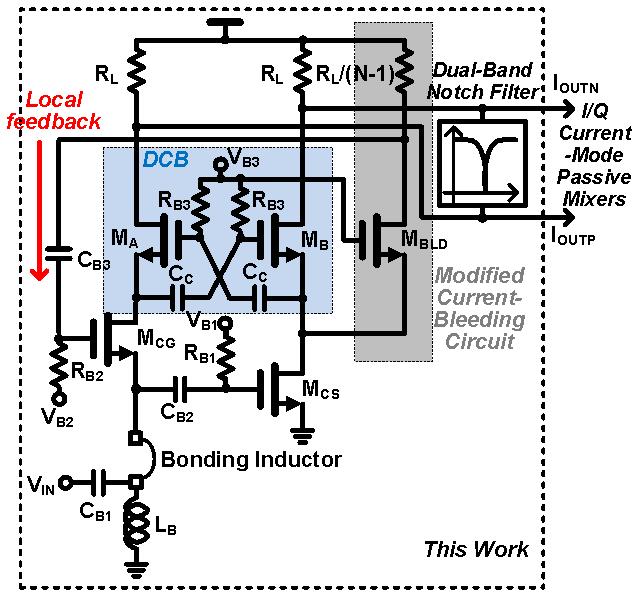### A. CG-CS LNTA with Local Feedback gm-boosting and Modified Current Bleeding Techniques

In the design of a low-noise and high-linearity broadband balun LNA, CG-CS balun LNAs are typically employed [3-9]. The 1:N CG-CS balun-LNA increases the g$_{m}$ of the CS amplifier by N times that of the CG amplifier, significantly reducing the noise contribution of the CS amplifier, thereby improving the noise figure (NF). However, output imbalance occurs due to the inherently unbalanced load. Recently, 1:N CG-CS balun-LNAs/LNTAs with local feedback g$_{m}$-boosting and modified current-bleeding techniques have been introduced, achieving output balance and an NF of less than 3 dB [7,9]. The proposed 1:5 CG-CS balun-LNTA adopts this LNA topology. Local feedback can be configured by connecting the drain of the current-bleeding transistor(M$_{BLD}$) to the gate of the CG transistor(M$_{CG}$) and modified current bleeding can be configured by R$_{L}$/(N-1) load resistance, (N-1) times the size of the current bleeding transistor(M$_{BLD}$) as shown in Fig. 1. The differential current balancer (DCB), composed of cascode amplifiers (M$_{A}$ and M$_{B}$) with cross-coupled capacitors (C$_{C}$), amplifies the difference between the output voltages of M$_{CG}$ and M$_{CS}$ and forces the output currents to become I$_{OUTP}$ = -I$_{OUTN}$ . Because the DCB improves the differential balance characteristic of the proposed LNTA, the input currents of the double-balanced mixer are balanced. Component values used in the LNTA design are as follows: R$_{L}$= 0.5 k${\Omega}$, N = 5, I$_{MCG}$ = 0.92 mA, I$_{MCS}$ = 4.6 mA, and I$_{MBLD}$ = 3.68 mA.

Local feedback improves the overall transconductance of the CG amplifier by closed-loop gain. The local feedback g$_{m}$-boosting balun-LNTA can perform input power matching and achieve a low NF with low power consumption. The current mirror circuit sets the dc bias currents of the CS and CG amplifiers. Cascode transistors improve the isolation between the input and output of LNTA. The CG transistor is biased by an external RF choke inductor, L$_{B}$. Because the inductor has no dc voltage drop, the over-drive voltages of the CG and CS transistors are the same. To obtain an S$_{11}$ value of less than ${-}$ 10 dB (over the frequency range 50 MHz-6 GHz), L$_{B}$ = 20 nH is selected.

The transconductance gain of the proposed balun-LNTA from the voltage source, V$_{S}$, to the differential output current, I$_{OUT}$, can be derived as follows.

##### (1)
$G_{m}=\frac{I_{OUT}}{V_{S}}=\frac{2R_{IN}}{R_{S}+R_{IN}}(1+A_{OpenLoop})g_{m}=\frac{2R_{IN}}{R_{S}+R_{IN}}Kg_{m}$,

where g$_{m}$ is the CG transistor transconductance; A$_{OpenLoop}$ = (N-1)g$_{m}$R$_{L}$/[(N-1) + g$_{m}$R$_{L}$ ]; and R$_{IN}$, R$_{S}$, and R$_{L}$ are the input, source, and load resistance, respectively. The input impedance of the following current-mode double-balanced passive mixer was assumed to be sufficiently lower than R$_{L}$. The NF of the proposed balun-LNTA can be approximately expressed as 

##### (2)
$NF_{dB}\cong 10\log \left[1+\frac{\gamma }{K}\frac{3}{(1+\chi )^{2}}+\frac{\gamma }{K}\frac{N\chi ^{2}}{(1+\chi )^{2}}\right]$,

where ${\gamma}$ is the noise parameter, ${\chi}$ = g$_{m}$R$_{L}$/(N-1). Moreover, ${\zeta}$ is given by

##### (3)
$\zeta =\left(\frac{(N-2)(1+\chi )}{N(1+\chi )+(N-2)(\chi -1/K)}\right)^{2}$.

### B. Dual-band Q-enhanced LC Notch Filter with Band-switchable Differential Inductor

The proposed dual-band Q-enhanced third-order LC notch filter is shown in Fig. 2. It has a negative-resistance (R) circuit to improve the Q-factor of the notch filter with the proposed band-switchable differential inductor. With this proposed inductor, the notch filter can support both low-band (LB) and mid-band (MB) frequencies in the 5G NR frequency-division duplexing (FDD) system.

##### Fig. 2. Proposed dual-band Q-enhanced LC notch filter with band-switchable differential inductor.}The layout of the proposed band-switchable differential inductor with a center tap is shown in Fig. 3. By switching the LB on and MB off, the entire differential inductor for LB operation can be utilized. By switching the LB on and LB off, some of the inductors for MB operation are disabled, thus reducing inductance. The metal width, metal spacing, and outer radius of the inductor were 15, 5, and 200 ${\mu}$m, respectively. The distance between the differential inductor and switches (SW$_{LB}$ and SW$_{MB}$) is 60 ${\mu}$m. The inductance and Q-factor of the designed band-switchable differential inductor were completely simulated including the routing line to switches through electromagnetic simulation using the Cadence EMX tool. As shown in Fig. 4, the inductance and Q-factor at LB and MB are approximately 8.96 nH/10 and 3.26 nH/12, respectively.

##### Fig. 3. Proposed band-switchable differential inductor with a center tap.}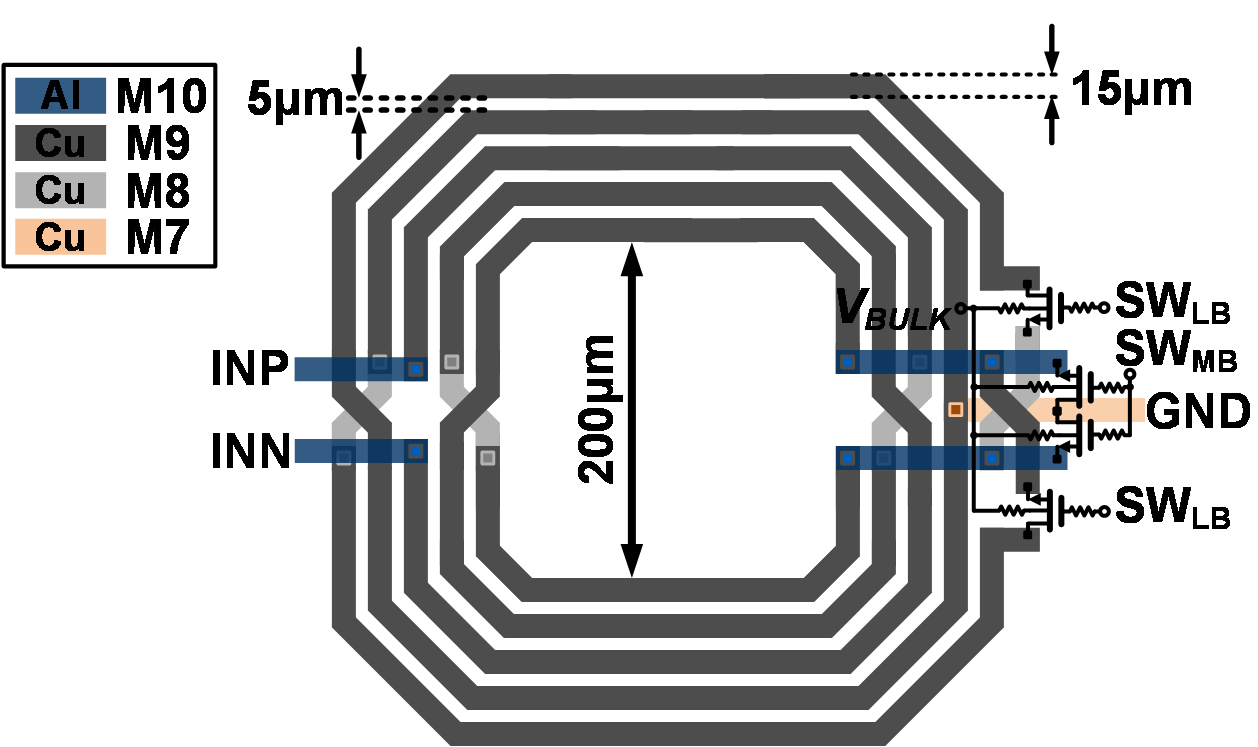##### Fig. 4. Simulated inductances and Q-factors of the band-switchable differential inductor at LB and MB.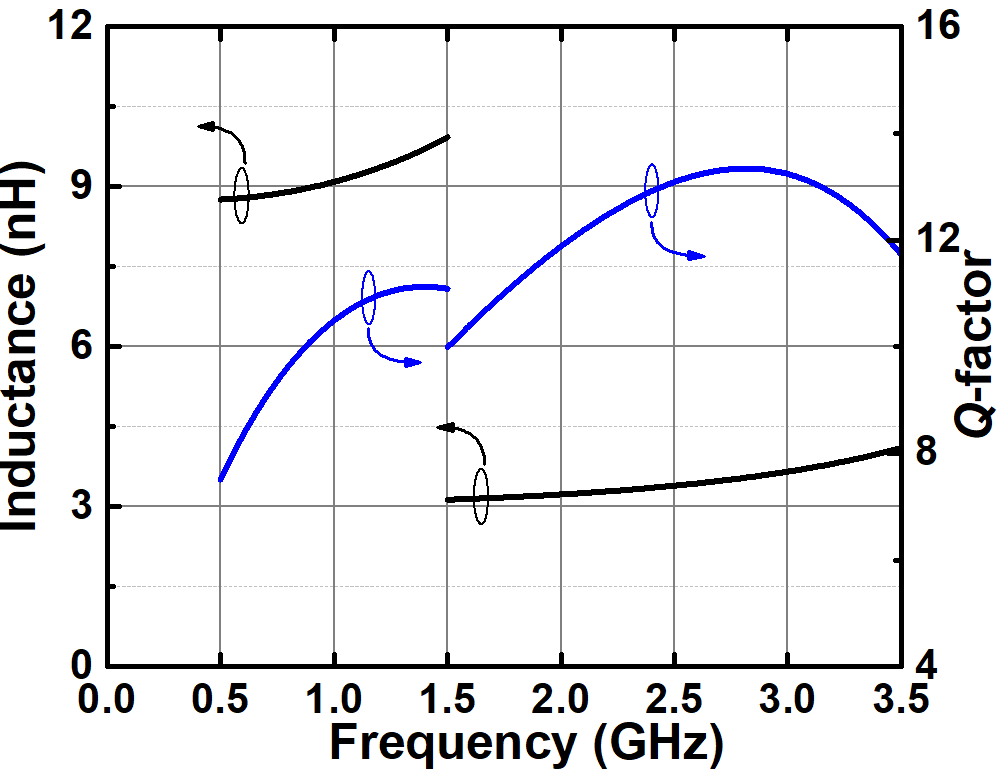The notch frequency of an LC notch filter can be tuned to the strong out-of-band blockers or TX leakage signals using 5-bit digitally controlled capacitor arrays (C$_{N1}$ and C$_{N2}$). The overall transconductance gain of LNTA with an embedded LC notch filter can be expressed as

##### (4)
$G_{mNotch}=G_{m}\frac{G_{m}Z_{N}}{\left(1+G_{m}Z_{N}\right)}$

where Z$_{N}$ is the equivalent impedance observed in the LC notch filter. It is given to the following:

##### (5)
$Z_{N}=\frac{1+s^{2}L_{N}(\frac{C_{N1}}{2}+C_{N2})}{\frac{sC_{N1}}{2}(1+s^{2}L_{N}C_{N2})}=\frac{1+s^{2}/\omega _{N}^{2}}{\frac{sC_{N1}}{2}(1+s^{2}/\omega _{P}^{2})}$,

where f$_{P}$ and f$_{N}$ are the pole and notch frequencies, respectively. In the passband frequency, G$_{mNotch}$ becomes G$_{m}$ and approaches zero near f$_{N}$. As known from (5), the third-order LC notch filter has a pole around the RX band, so that a flat frequency characteristic can be obtained without droop around the RX band . It prevents the gain and NF degradation at the RX band caused by the notch filtering. The finite Q-factor of L$_{N}$ and the switched-on resistance of the capacitor arrays of C$_{N1}$ and C$_{N2}$ reduce the overall Q-factor of the LC notch filter, consequently degrading notch selectivity. To improve the notch depth, the negative-R circuit shown in Fig. 2 is used .

## III. SIMULATION RESULTS

The proposed low-noise broadband balun-LNTA with an embedded Q-enhanced third-order LC notch filter for sub-6 GHz 5G NR cellular applications was designed in a 65-nm CMOS process. The layout of the balun-LNTA is illustrated in Fig. 5. The total active die area without the bond pads is 0.55 mm$^{2}$. To verify the performance of the designed balun-LNTA, post-layout simulations were performed.

##### Fig. 5. Layout of the designed balun-LNTA.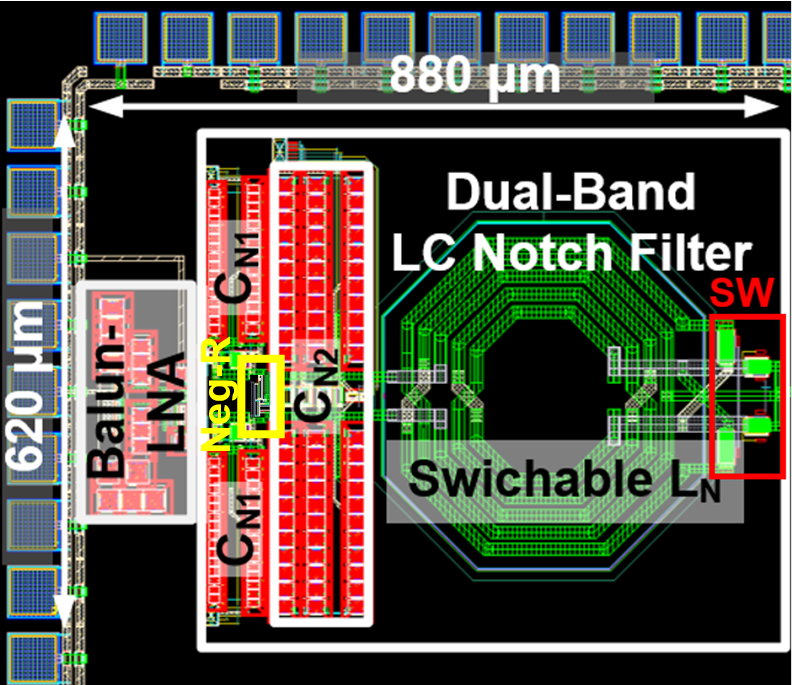The simulated S$_{11}$ is shown in Fig. 6. The balun-LNTA has an S$_{11}$ value of less than ${-}$10 dB (over the frequency range 50 MHz-6 GHz). Fig. 7 shows the simulated transconductance gain of the balun-LNTA versus RF frequencies with and without the negative-R circuit. The LNTA obtains a maximum transconductance gain of 44 mS at 500 MHz and 3-dB bandwidth of 6.24 GHz. The notch frequency can be adjusted to the TX frequency or strong interferer frequency of the operating band by tuning the inductance and capacitance of the third-order LC notch filter. Because of the negative-R circuit, the simulated notch depth can exceed 15 dB throughout the operating frequencies of the sub-6-GHz 5G NR. The simulated gain and phase mismatches of differential output currents are described in Fig. 8. In the entire frequency range, the gain and phase mismatches are less than 0.5 dB and 2$^{\circ}$, respectively. The simulated NF of the balun LNTA with the loading effect of the input impedance of the following current-mode double-balanced passive mixer is shown in Fig. 9. The figure indicates that the simulated NF is less than 4.8 dB at a maximum frequency of 6 GHz, and the minimum NF is 2.38 dB. The simulated in-band input-referred third-order intercept point (IIP3) is depicted in Fig. 10. The two-tone test conditions for the IIP3 are f$_{1}$ = f$_{RXLO}$ + 1 MHz, f$_{2}$ = f$_{RXLO}$ + 1.1 MHz, and p$_{f1}$ = p$_{f2}$ = ${-}$40 dBm, where f$_{RXLO}$ is the RX LO frequency. The simulated in-band IIP3 exceeds ${-}$6.1 dBm throughout the band.

##### Fig. 7. Simulated frequency response of the notch filtered LNTA with and without the negative-R circuit.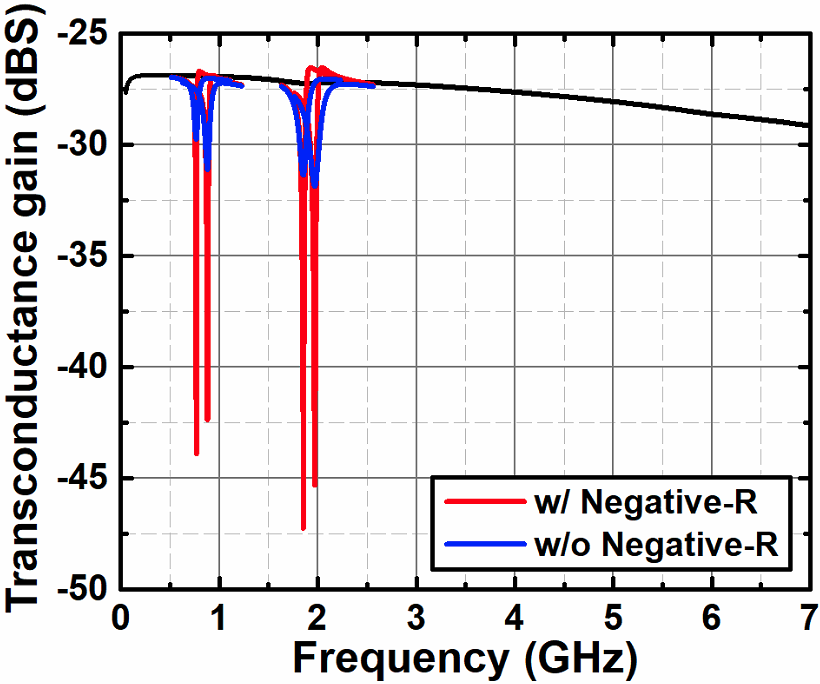##### Fig. 8. Simulated gain and phase mismatches.##### Fig. 10. Simulated in-band IIP3.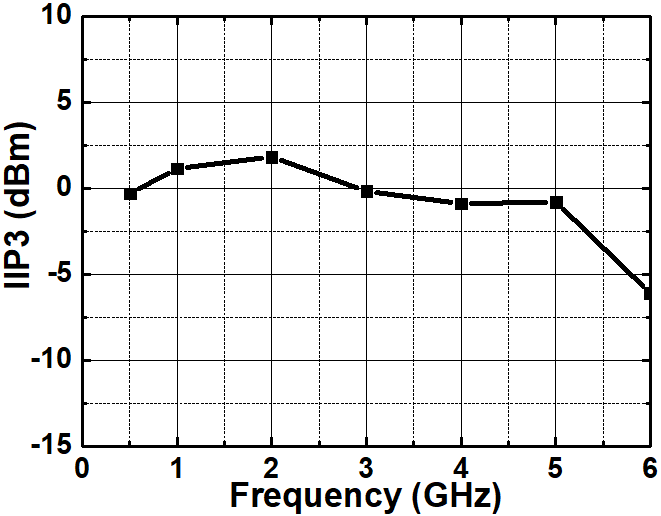Table 1 summarizes and compares the performances of the designed balun-LNTA with previous state-of-the-art balun-LNAs. The proposed balun-LNTA provides a broadband frequency response with a minimum NF of 2.38 dB and a transconductance gain of 44 mS with balanced differential output currents. It is the first balun-LNTA with an embedded dual-band LC notch filter that can sufficiently reject TX leakage signals and out-of-band blockers.

##### Table 1. Simulated Performance Summaries of the Proposed Balun-LNTA and Comparison with Previous Balun-LNAs
 Architecture Process Balanced Load Balun Function RF Filtering Frequency [GHz] Gain [dB] / Gm [mS] NF [dB] IIP3 [dBm] IIP2 [dBm] Power [mW] Supply [V] Area [mm$^{2}$] JSSC 2008  CG-CS LNA 65-nm CMOS NO YES NO 0.2-5.2 13-15.6 / - 2.9-3.5 0 22 21 1.2 0.01 TCAS-I 2010  CG-CS LNA + Local FB 0.13-${\mathrm{\mu}}$m CMOS NO YES NO 0.2-3.8 16-19 / - 2.8-3.4 -4.2 NR† 5.7 1 0.025 TMTT 2012  CG-CS LNA + Local FB 0.13-${\mathrm{\mu}}$m CMOS NO YES NO 0.1-2 13.6-16.6 / - 3.8-5 2 24 3 1.2 0.075 TCAS-I 2019  CG-CS LNA + Modified CBLD* 65-nm CMOS YES YES NO 0.05-1 24-30 / - 2.3-3.3 -4.1 20.6 19.8 2.2 0.045 TCAS-I 2020  CG-CS LNA + Local FB + Modified CBLD 65-nm CMOS YES YES NO 0.05-1.3 24 - 27.5 / - 2.3-3 -2.2 19.6 5.7 1 0.046 TMTT 2022 ** GB‡ N-Path Balun-LNA 65-nm CMOS YES YES YES (BPF) 0.7-2.2 21.9-26.8 /- 2.9-3.8 11.4-19.1⁑ NR† 10.8 1 NR† This Work** CG-CS LNA + Local FB + Modified CBLD +LC Notch Filter 65-nm CMOS YES YES YES (Notch) 0.05-6.24 - / 31.1-44 2.38-4.8 1.82 49.9 5.9 1 0.55
†NR: not reported *CBLD: current bleeding technique ** Post-layout simulation results ‡GB: gain-boosted ⁑ Out-of-band IIP3

## IV. CONCLUSION

A broadband low-noise noise-cancelling balun-LNTA with an embedded dual-band Q-enhanced third-order LC notch filter is designed to implement a blocker-tolerant receiver for 5G NR sub-6 GHz cellular applications. To design the dual-band LC notch filter, a band-switchable differential inductor with a center tap was proposed. The proposed balun-LNTA can reject unwanted blockers and strong TX-leakage signals in the 5G NR FDD bands, thus relaxing the linearity requirement of the following mixer. It also achieves low NF and broadband characteristics by employing a 1:5 CG-CS balun-LNTA topology with local feedback g$_{m}$-boosting and current bleeding techniques. Based on the simulation results, it obtains a minimum NF of 2.38 dB, a maximum transconductance gain of 44 mS and a 3-dB bandwidth of 6.24 GHz. Moreover, its blocker rejection ratio for the LB and MB of the 5G NR exceeds 15 dB.

## ACKNOWLEDGMENTS

This work was supported by the Basic Science Research Program through the National Research Foundation of Korea (NRF) funded by the Ministry of Education under Grant NRF-2018R1D1A1B07042804. The chip fabrication and EDA tool were supported by the IC Design Education Center (IDEC), Korea.

## References

1
Lee J., Han S., Lee J., Kang B., Bae J., Jang J., Oh S., Ahn S., Kang S., Bui Q., Son K., Lim H., Jeong D., Ni R., Zuo Y., Jong I., Yao C., Heo S., Cho T., Kang I., Dec. 2019, A sub-6GHz 5G new radio RF transceiver supporting EN-DC with 3.15Gb/s DL and 1.27Gb/s UL in 14nm FinFET CMOS, IEEE J. Solid-State Circuits, Vol. 54, No. 12, pp. 3541-35522
Tsai M., Yang S., Yu C., Chen P., Wu T., Hassan M., Chen C., Wang C., Huang Y., Hung L., Chiu W., Lin A., Lin B., Werquin A., Lin C., Chen Y., Tsai J., Fu Y., Tenbroek B., Chiu C., Lee Y., Dehng G., Feb. 2020, A 12nm CMOS RF transceiver supporting 4G/5G UL MIMO, in IEEE Int. Solid-State Circuits Conf. Tech. Dig., San Francisco, CA, pp. 176-1773
Blaakmeer S. C., Klumperink E. A. M., Leenaerts D. M. W., Nauta B., Jun. 2008, Wideband balun-LNA with simultaneous output balancing, noise canceling and distortion-canceling, IEEE J. Solid-State Circuits, Vol. 43, No. 6, pp. 1341-13504
Wang H., Zhang L., Yu Z., Aug. 2010, A Wideband inductorless LNA with local feedback and noise cancelling for low-power low-voltage applications, IEEE Trans. Circuits Syst. I, Reg. Papers, Vol. 57, No. 8, pp. 1993-20055
Kim J., Silva-Martinez J., Sept. 2012, Wideband inductorless balun-LNA employing feedback for low-power low-voltage applications, IEEE Trans. Microw. Theory Tech., Vol. 60, No. 9, pp. 2833-28426
Kim S., Kwon K., Feb. 2019, A 50 MHz-1 GHz 2.3-dB NF noise-cancelling balun-LNA employing a modified current-bleeding technique and balanced Loads, IEEE Trans. Circuits Syst. I, Reg. Papers, Vol. 66, No. 2, pp. 546-5547
Kim S., Kwon K., Dec. 2020, Broadband balun-LNA employing local feedback g$_{m}$-boosting technique and balanced loads for low-power low-voltage applications, IEEE Trans. Circuits Syst. I, Reg. Papers, Vol. 67, No. 12, pp. 4631-46408
Shin D., Lee K., Kwon K., A blocker-tolerant receiver front end employing dual-band N-path balun-LNA for 5G new radio cellular applications, Early access in IEEE Trans. Microw. Theory Tech9
Lee D., Kwon K., Nov. 2021., A blocker-tolerant balun-LNTA employing LC notch filter for 5G new radio cellular applications, in The Sixth International Conference on On Consumer Electronics Asia (IEEE/IEIE ICCE-Asia 2021), Kangwon, Korea10
Kwon K., Kim S., Son K., Nov. 2018, A hybrid transformer-based CMOS duplexer with a single-ended notch-filtered LNA for highly integrated tunable RF front-ends, IEEE Microw. Wireless Compon. Lett., Vol. 28, No. 11, pp. 1032-103411
Vallese A., Bevilacqua A., Sandner C., Tiebout M., Gerosa A., Neviani A., Feb. 2009, Analysis and design of an integrated notch filter for the rejection of interference in UWB systems, IEEE J. Solid-State Circuits, Vol. 44, No. 2, pp. 331-34312
Darabi H., Dec. 2007, A blocker filtering technique for SAW-less wireless receivers, IEEE J. Solid-State Circuits, Vol. 42, No. 12, pp. 2766-277313
Lee D., Kwon K., CMOS channel-selection LNA with a feedforward N-path filter and calibrated blocker cancellation path for FEM-less cellular transceivers, Early access in IEEE Trans. Microw. Theory Tech.14
Qi G., Liempd B., Mak P., Martins R., Craninckx J., May 2018, A SAW-less tunable RF front end for FDD and IBFD combining an electrical-balance duplexer and a switched-LC N-path LNA, IEEE J. Solid-State Circuits, Vol. 53, No. 5, pp. 1431-144215
Kim T., Lee D., Kwon K., March. 2020, CMOS channel-selection low-noise amplifier with high-Q RF band-pass/band-rejection Filter for highly integrated RF front-ends, IEEE Microw. Compon. Lett., Vol. 30, No. 3, pp. 280-28316
Luo C., Gudem P., Buckwalter J., Apr. 2016, A 0.4-6-GHz 17-dBm B1dB 36-dBm IIP3 channel-selecting low-noise amplifier for SAW-less 3G/4G FDD diversity receivers, IEEE Trans. Microw. Theory Techn., Vol. 64, No. 4, pp. 1110-112117
Mak P., Martins R., Sept. 2011, A 0.46-mm2 4-dB NF unified receiver front-end for full-band mobile TV in 65-nm CMOS, IEEE J. Solid-State Circuits, Vol. 46, No. 9, pp. 1970-1984Donggu Lee received B.S. and M. S. degrees from the Department of Electronics Engineering, Kangwon National University, Chuncheon, South Korea, in 2019 and 2021, respectively. He is currently working toward the Ph. D. degree in the Department of Electronics Engineering, Kangwon National University, Chuncheon, South Korea. His research interests include CMOS mmWave/RF/analog integrated circuits and RF system design for wireless communications. D. Lee was a recipient of KIPO (Korean Intellectual Property Office) Commissioner award and ADT special award for Korea Semiconductor Design Competition in 2018 and 2019, and 3rd award for 11th ETNEWS ICT best paper award in 2019.

Kuduck Kwon received the B.S. and Ph.D. degrees in Electrical Engi-neering and Computer Science from Korea Advanced Institute of Science and Technology (KAIST), in Daejeon, Korea, in 2004 and 2009, respectively. His doctoral research concerned digital TV tuners and dedicated short-range communication (DSRC) systems. From 2009 to 2010, he was a Post-Doctoral Researcher with KAIST, where he studied a surface acoustic wave (SAW)-less receiver and developed RF transceivers for DSRC applications. From 2010 to 2014, he was a Senior Engineer with Samsung Electronics Co. LTD., Suwon, Korea, where he was involved in studying software-defined receiver and developing silicon tuner and cellular RFICs. In 2014, he joined the Department of Electronics Engineering, Kangwon National University, Chuncheon, Korea, where he is currently an Associate Professor. His research interests include CMOS mmWave/RF/analog integrated circuits and RF system design for wireless communications.